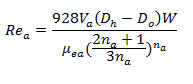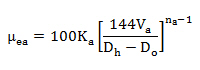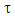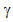## Reynold Number for Drilling Hydraulics

Reynold number is the important figure because it demonstrates the flow regimes of drilling mud as laminar, transition or turbulent flow in annulus of the wellbore. In order to correctly calculate the Reynolds number, you need to use the effective viscosity, µea, which we already discuss about it from the previous topic.

The following equation is for Reynolds number in the annulus.Where:

Rea = Reynold Number in the annulus

Va = Annular velocity, ft/min

Dh = Diameter of wellbore, inch

Do = Outside Diameter of tubular, inch

W = mud weight, ppg

µea = effective viscosity in the annulus, centi-poise

na = power law constant

## Effective Viscosity Calculation

The viscosity of drilling mud will change with a change in the shear rate because in some degree drilling fluids are shear-thinning. Because of its nature of drilling mud, people create the new term of viscosity called “Effective Viscosity” to compensate the change in shear rate of viscosity. By definition, the effective viscosity means the viscosity of Newtonian fluid that gives the same shear stress at the same shear rate.

The following equation is the effective viscosity equation.## Power Law Constants (n and K) Calculation

Many types of drilling mud are classified as non-Newtonian fluid which is the behavior between Newtonian fluid model and Bingham Plastic model. The relationship between shear rate and shear stress is defined by the power law model shown below:Where= shear rate

K = consistency factor= shear rate

n = flow behavior index

## Annular Velocity and Its Importance to Drilling Hydraulics

Annular velocity is the speed of fluid moving up the annulus and it must be high enough to transport cuttings generated while drilling from the wellbore. However, if the annular velocity is too high, it can create hole wash out and excessive equivalent circulating density.

When the drilling mud is circulated through a system, the moving speed is lower at location where the cross section area is bigger. Conversely, when the fluid flows through the small cross section area, the annular velocity at that point is higher.

The cross section area around drill collar and BHA has the smallest area so the annular velocity is the highest.(Annular velocity around drill collar and BHA)

## System Constrain for Drilling Hydraulics

When we get the optimum flow rate which match your optimization objective, you must check the system constrains such as

• Deliverability of mud pumps is enough, isn’t it?

• The optimum flow rate is within the operating range of down hole tools, isn’t it?

• The maximum annular velocity is exceeded, isn’t it?

• The equivalent circulating density can cause formation fracture, can’t it?

• The flow rate is below the critical flow rate, isn’t it?

In the following articles, we will have more in depth details regarding system constrains for drilling hydraulics. The topics that we will discuss are as follows:

• Annular velocity and its importance on drilling hydraulics

• Power Law constant determination

• Effective viscosity

• Reynolds number

• Flow regime and critical Reynolds numbers

• Critical flow rate

We also have an intention to demonstrate step-by-step calculation to show you all calculation because only equations are not good enough to get all the pictures.

Reference:Drilling Hydraulic Books